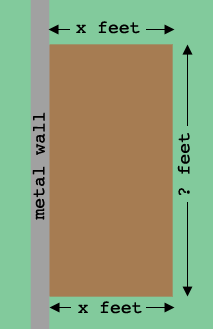SEARCH HOMEMath Central Quandaries & QueriesQuestion from Miguel: Stephanie has 60 feet of metal fencing material to only fence 3 sides of a rectangular patio. A tall metal wall serves as the fourth side of the rectangle. write a function for the area the garden (A) in terms of width in feet (x) What measures of the width would give an area of at least 150 feet?Hi Miguel,

I think you mean "area of at least 150 SQUARE feet".In my diagram the patio has 2 sides of length $x$ feet each. There are 60 feet of fencing. How long is the third side? What is the area of the patio in square feet? Write a mathematical expression for the statement that the area is less than or equal to 150 square feet and rewrite this expression in the form $a x^2 + b x + c \leq 0.$

To solve this inequality for $x$ I would first solve $a x^2 + b x + c = 0$ for $x.$ Use these roots to sketch the graph of $y = a x^2 + b x + c .$ For what values of $x$ does the graph lie below the X-axis?

PennyMath Central is supported by the University of Regina and The Pacific Institute for the Mathematical Sciences.